# 4-3i 11. (8 pts) Forz 4-3i much as possible, writin work. i and w 7- i,...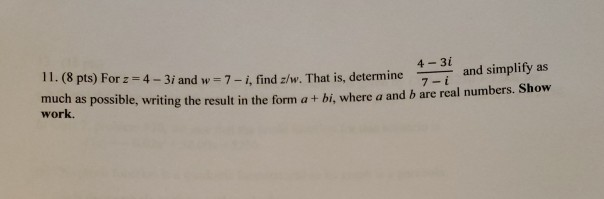4-3i 11. (8 pts) Forz 4-3i much as possible, writin work. i and w 7- i, find z/w. That is, determineand simplify as g the result in the form a + bi, where a and b are real numbers. Show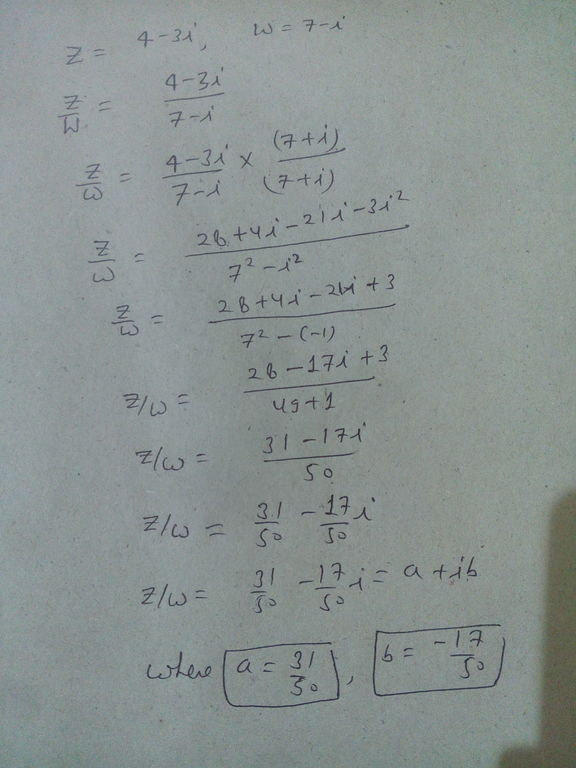#### Earn Coin

Coins can be redeemed for fabulous gifts.

Similar Homework Help Questions
• ### and z2 = 1 1 + 3i 3-i a) Given that zı = find z such...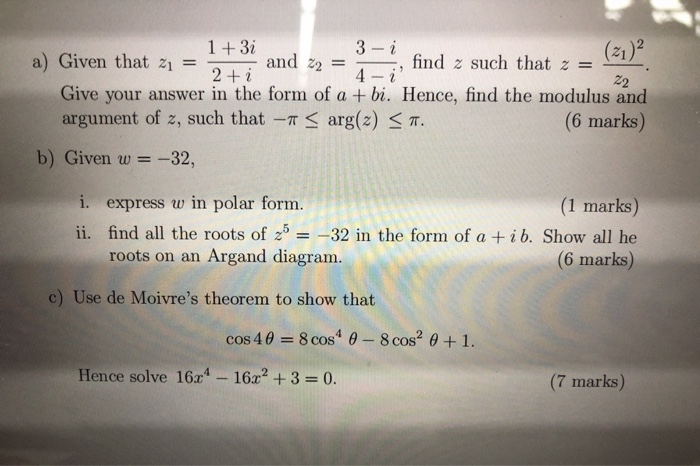and z2 = 1 1 + 3i 3-i a) Given that zı = find z such that z = 2 + i 4- ¿ 22 Give your answer in the form of a + bi. Hence, find the modulus and argument of z, such that -- < arg(2) < 7. (6 marks) b) Given w = = -32, i. express w in polar form. (1 marks) ii. find all the roots of 2b = -32 in the form of a...

• ### 2iz-1 If f(2) = 27251 2-3i a) Find and simplify f'(z) b) Find f'(1 + i)...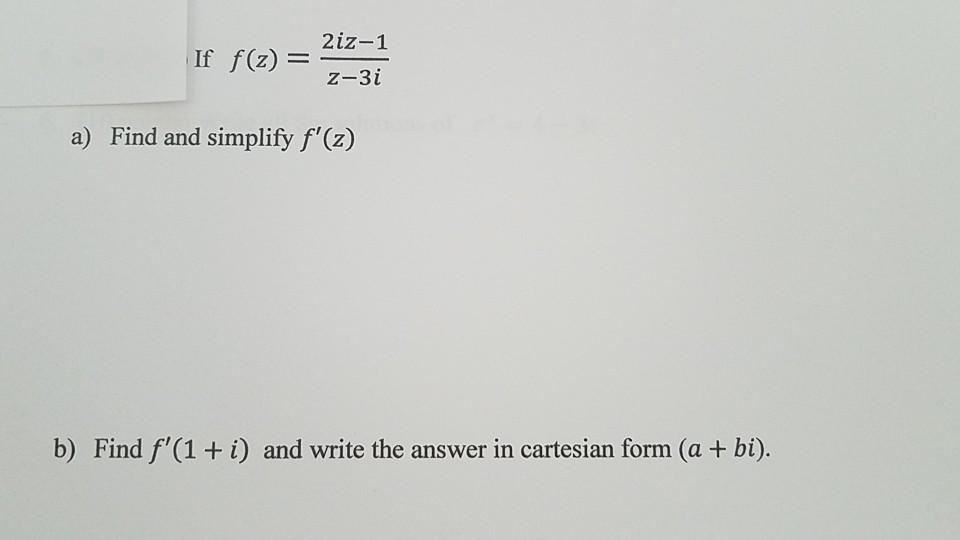2iz-1 If f(2) = 27251 2-3i a) Find and simplify f'(z) b) Find f'(1 + i) and write the answer in cartesian form (a + bi).

• ### (1 point) Suppose pP(z) 5x2+ z+3 (a) Simplify as much as possible: p(-1)=7 help (numbers) (b)...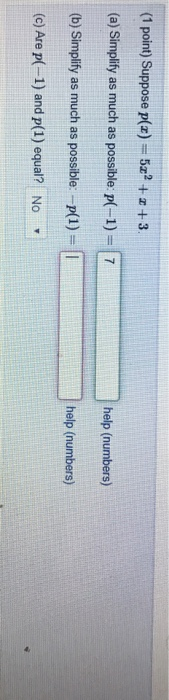(1 point) Suppose pP(z) 5x2+ z+3 (a) Simplify as much as possible: p(-1)=7 help (numbers) (b) Simplify as much as possible: -p(1)= | help (numbers) (c) Are p(-1) and p(1) equal? No (1 point) Suppose pP(z) 5x2+ z+3 (a) Simplify as much as possible: p(-1)=7 help (numbers) (b) Simplify as much as possible: -p(1)= | help (numbers) (c) Are p(-1) and p(1) equal? No

• ### Find w=a +bi = VE , where a and b are real numbers. 7601 - 11...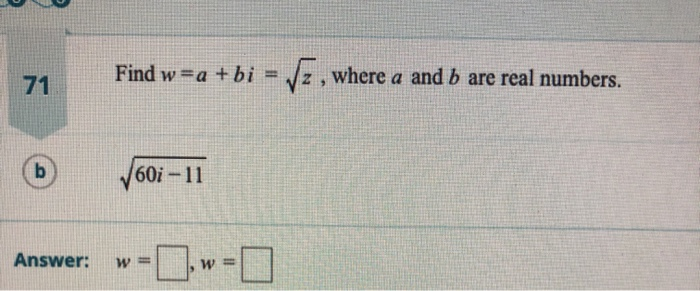Find w=a +bi = VE , where a and b are real numbers. 7601 - 11 Answer: w= w=

• ### Perform the indicated operation and write the answer in the form a +bi, where a and...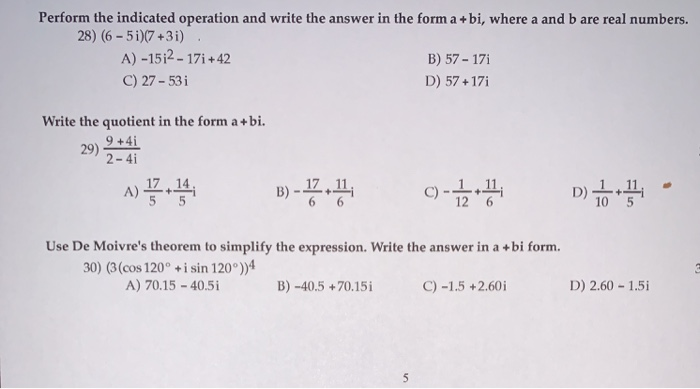Perform the indicated operation and write the answer in the form a +bi, where a and b are real numbers. 28) (6-5i)(7 +3i) A) -15;2 - 171 +42 B) 57 - 171 C) 27 - 531 D) 57 +171 Write the quotient in the form a +bi. 9 +41 29) 2-4i B) -1.4, C) -1 5 Use De Moivre's theorem to simplify the expression. Write the answer in a +bi form. 30) (3 (cos 120° + i sin 120°))4 A)...

• ### Please solve #3 and #4. Show work if possible thanks! 2. Assume variables represent positive real...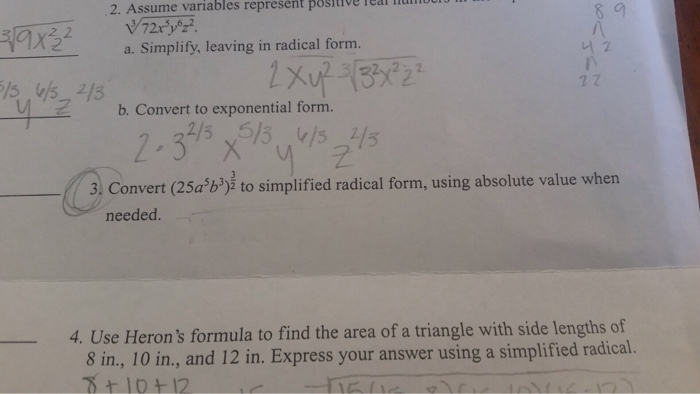Please solve #3 and #4. Show work if possible thanks! 2. Assume variables represent positive real li a. Simplify, leaving in radical form. 너2 2 7 b. Convert to exponential form. enseryoa 3, Convert (25ab) to simplified radical form, using absolute value when needed. 4. Use Heron's formula to find the area of a triangle with side lengths of 8 in, 10 in, and 12 in. Express your answer using a simplified radical. 10+12

• ### Include all relevant work please. 8. Find the general polynomial function of lowest possible degree with...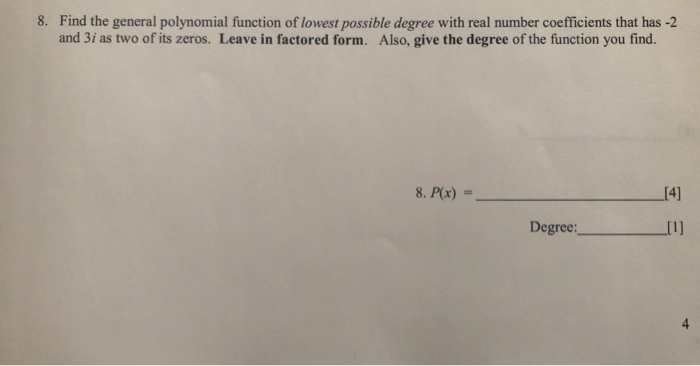Include all relevant work please. 8. Find the general polynomial function of lowest possible degree with real number coefficients that has -2 and 3i as two of its zeros. Leave in factored form. Also, give the degree of the function you find. 8. P(x)  Degree:

• ### AB 31) Find the eigenvalues and eigenspaces of the matrix-4 0 4 Hint: One way to...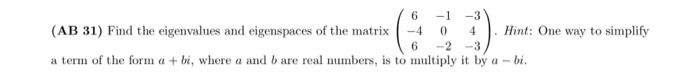AB 31) Find the eigenvalues and eigenspaces of the matrix-4 0 4 Hint: One way to simplify 6 -2-3 a term of the form a +bi, where a and b are real numbers, is to multiply it by a -bi

• ### 8. If ū= 8î - 159 and v = -3i - 4ſ and w = 12...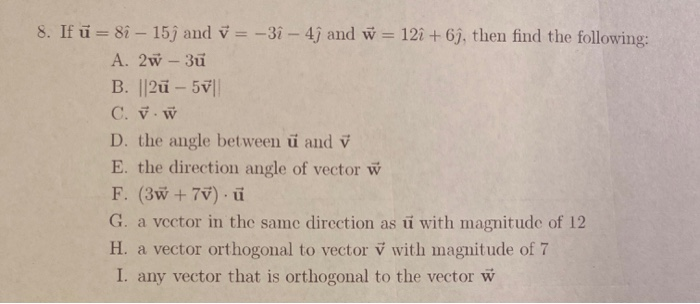8. If ū= 8î - 159 and v = -3i - 4ſ and w = 12 + 69, then find the following: A. 2w - 3ū B. ||2u - 57 C. v. W D. the angle between ü and v E. the direction angle of vector w F. (3 +70).ü G. a vector in the same direction as ū with magnitude of 12 H. a vector orthogonal to vector v with magnitude of 7 I. any vector that is orthogonal...

• ### 3. (8 points each) Find the derivative of each function. Show as much work as possible....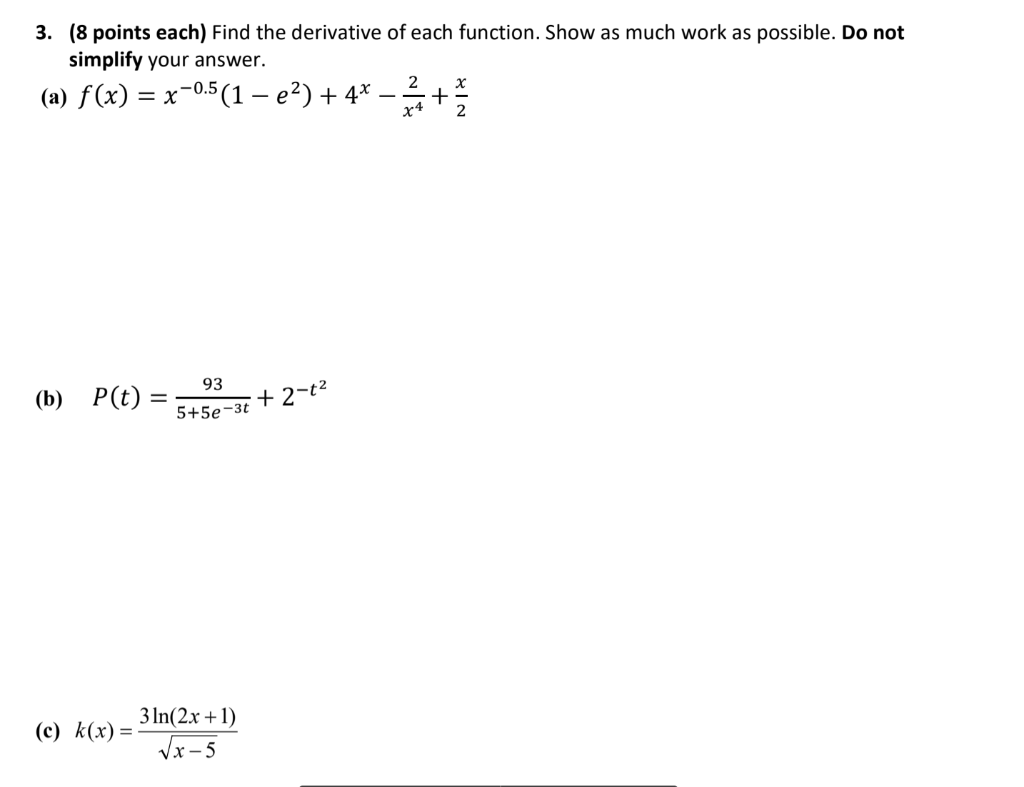3. (8 points each) Find the derivative of each function. Show as much work as possible. Do not simplify your answer. 2 (a) f(x) = x-0.5(1 – e2) + 4* + x4 x 2 93 (b) P(t) = + 2-t2 5+5e-3t (c) k(x) = 3 ln(2x +1) VX-5

Free Homework App